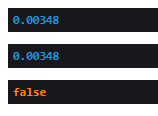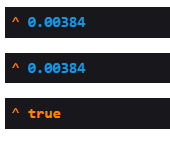# Problem on check condition on laravel php

I have a problem on my laravel code. I want to check condition and show up using dd my code here.

```\$ca = floatval(\$sw->terminal_ca); // \$sw->terminal_ca from postgresql type number(8,6) = 0.003480
\$max_ca = \$this->terminal_ca*(1+(\$this->tolerance/100)); // \$this->terminal_ca from postgresql type number(16,4) = 0.0029
// \$this->tolerance from postgresql type number(16,4) = 20.0000

dd(
\$ca,
\$max_ca,
(\$ca <= \$max_ca));```

but my result herenot acceptable.
it should be true.
What’s wrong on my code?
How can I solve it?

Contents

### Method 1

I have given static values in the controller and it’s working fine.
Kindly check your type in the database from where you are fetching data dynamically.### Method 2

I round it before comparison is ok now. Thank @El_Vanja

```\$ca = round(\$ca, 6);
\$min_ca = round(\$min_ca, 6);```